• ## java实现开根号

万次阅读 2017-08-22 20:46:51
java开根号
主要思路
通过牛顿迭代法计算平方根。来吧，还是看代码ba..
    private static double sqrt(double c) {
if (c < 0)
return Double.NaN;

double jingdu = 1e-15;
double t = c;
while (Math.abs(c - t * t) > jingdu)
t = (c / t + t) / 2.0;// 迭代公式
return t;
}
改进算法。。。
    public static double sqrt(double m) {
double i = 0;
int k = 0;
while ((i * i) <= m)
i += 0.1;
for (int j = 0; j < 10; j++) {
i = (m / i + i) / 2;
}
return i;
}
展开全文算法
• ## Java实现开根号

千次阅读 2018-09-30 02:21:47
Java实现开根号 二分法 static float sqr(float x){ float max = x; float min = 0; float mid=(min+max)/2; while (true){ if (Math.abs(x-mid*mid)&amp;lt;0.002) return mid;...
Java实现开根号
二分法
   static float sqr(float x){
float max = x;
float min = 0;
float mid=(min+max)/2;
while (true){
if (Math.abs(x-mid*mid)<0.002) return mid;

if(mid*mid>x)
{
max=mid;
}
else if(mid*mid<x)
{
min=mid;
}

mid=(min+max)/2;
}

}

牛顿法
牛顿迭代法（Newton’s method）又称为牛顿-拉夫逊方法（Newton-Raphson method），它是牛顿在17世纪提出的一种在实数域和复数域上近似求解方程的方法。多数方程不存在求根公式，因此求精确根非常困难，甚至不可能，从而寻找方程的近似根就显得特别重要。方法使用函数f(x)的泰勒级数的前面几项来寻找方程f(x) = 0的根。牛顿迭代法是求方程根的重要方法之一，其最大优点是在方程f(x) = 0的单根附近具有平方收敛，而且该法还可以用来求方程的重根、复根。另外该方法广泛用于计算机编程中。
设r是f(x) = 0的根，选取x0作为r初始近似值，过点（x0,f(x0)）做曲线y = f(x)的切线L，L的方程为y = f(x0)+f’(x0)(x-x0)，求出L与x轴交点的横坐标 x1 = x0-f(x0)/f’(x0)，称x1为r的一次近似值。
过点（x1,f(x1)）做曲线y = f(x)的切线，并求该切线与x轴交点的横坐标 x2 = x1-f(x1)/f’(x1)，称x2为r的二次近似值。重复以上过程，得r的近似值序列，其中x(n+1)=x(n)－f(x(n))/f’(x(n))，称为r的n+1次近似值，上式称为牛顿迭代公式。
    static float Newton_sqr(float x){
float k = 1;
while (Math.abs(k*k-x)>1e-5){
k=(k+x/k)/2;
}
return k;
}

参考
https://blog.csdn.net/huaqianmian/article/details/51279627?utm_source=copy
展开全文• java实现开根号的运算 如果某个数字正好可以开根号为2个整数，例如1,4,9等，那就很简单了。 如果某个数字不可以正好开根号为2个整数，而且要保留几位精度，例如：2,3,5等，我们该怎么办呢？？？？？ 首先我们可以...
今天我们来看一下这道题，java如何实现开根号的运算。大家都知道，java,c++,python等都有直接的函数可以给我们用，但是面试官这个时候让我们手撕源码，这个时候怎么做呢，来，不要慌！！！如果某个数字正好可以开根号为2个整数，例如1,4,9等，那就很简单了。
如果某个数字不可以正好开根号为2个整数，而且要保留几位精度，例如：2,3,5等，我们该怎么办呢？？？？？
首先我们可以把这个数字分成整数部分和小数部分，分别计算。
例如√5≈2.236  我们可以先算出整数部分为2，然后在根据保留几位精度，去计算小数部分。依次计算十分位、百分位和千分位等，然后把整数位+十分位+百分位+千分位+。。。，结果就是我们想要的结果了。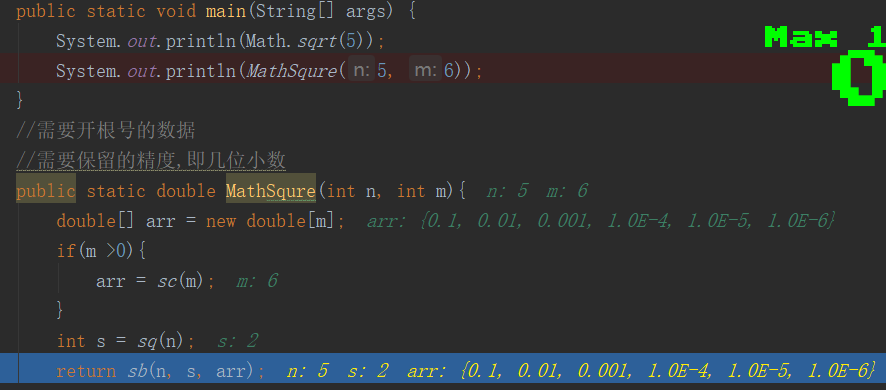来看代码
import java.math.BigDecimal;

/**
* @Auther: liuhaidong
* Data: 2020/4/21 0021、20:55
* Description:
* @version: 1.0
*/
public class Test6 {
public static void main(String[] args) {
System.out.println(Math.sqrt(5));
System.out.println(MathSqure(5, 6));
}
//需要开根号的数据
//需要保留的精度,即几位小数
public static double MathSqure(int n, int m){
double[] arr = new double[m];
if(m >0){
arr = sc(m);
}
int s = sq(n);
return sb(n, s, arr);
}
/**
* 计算要保留几位小数
* @param m
* @return
*/
public static double[] sc(int m){
double[] arr = new double[m];
int num = 0;
while(num != m){
double f = 1;
for(int i=0;i<=num;i++){
f = f*10;
}
arr[num] = 1/f;
num++;
}
return arr;
}
/**
* 计算整数位
* @param n
* @return
*/
public static int sq(int n){
if( n == 1){
return 1;
}
int tmp = 0;
for(int i=1;i<=n/2+1;i++){
if(i*i == n){
tmp = i;
break;
}
if(i*i > n){
tmp = i-1;
break;
}
}
return tmp;
}

/**
* 开根号
* @param n
* @param j
* @param arr
* @return
*/
public static double sb(int n, double j, double[] arr){
double tmp = j;
for(int p=0;p<arr.length;p++){
if(p>0){
j = tmp;//计算过后的值（整数位+小数位的和，赋值给j，下面继续运算）
}
for(int i=1;i<=9;i++){//小数位只有九位{0.1,0.2,0.3,0.4,0.5,0.6,0.7,0.8,0.9}
tmp = i*arr[p]+j;//i*arr[p],相当于每次加0.1,0.2 ...
if(tmp*tmp == n){
return tmp;
}
if(tmp*tmp >n){
//避免丢失精度
BigDecimal c1 = new BigDecimal(Double.toString(tmp));
BigDecimal c2 = new BigDecimal(Double.toString(arr[p]));
tmp = c1.subtract(c2).doubleValue();
break;
}
}
}
return tmp;
}
}

思路继续讲解。
小于2.3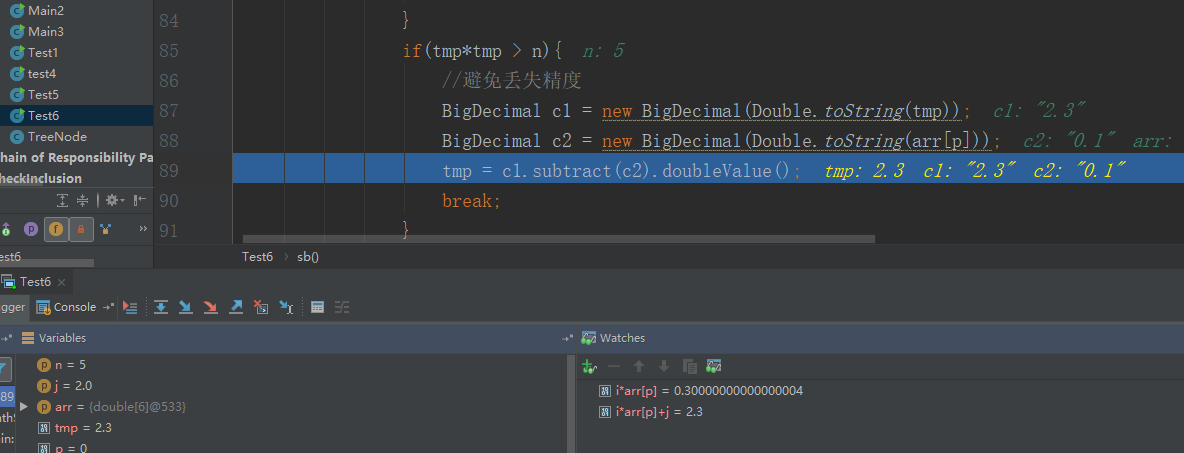把2.2记录在这里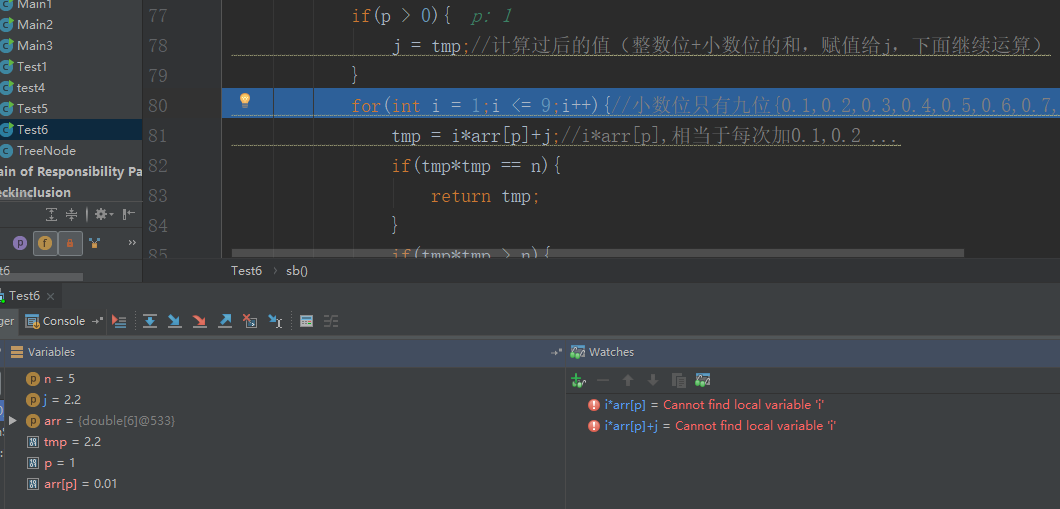小于2.24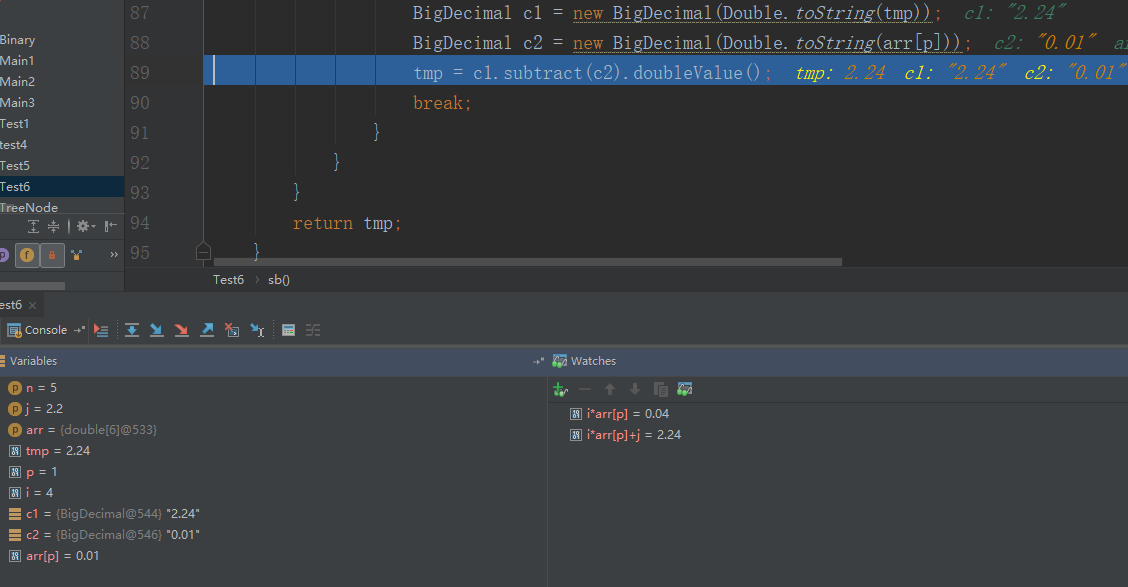记录2.23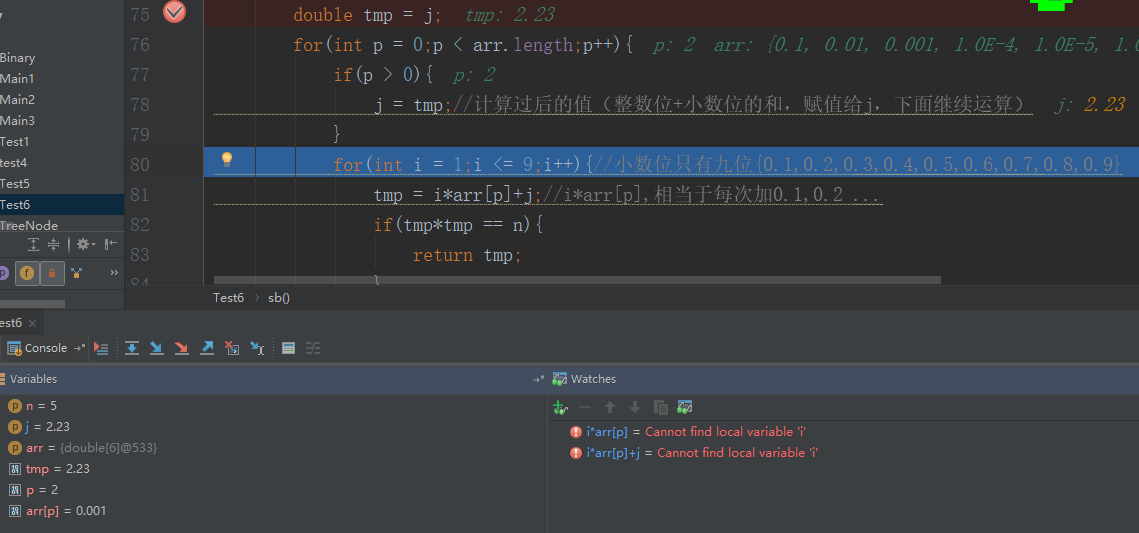依次这样做。本公众号分享自己从程序员小白到经历春招秋招斩获10几个offer的面试笔试经验，其中包括【Java】、【操作系统】、【计算机网络】、【设计模式】、【数据结构与算法】、【大厂面经】、【数据库】期待你加入！！！
1.计算机网络----三次握手四次挥手 2.梦想成真-----项目自我介绍 3.你们要的设计模式来了 4.震惊！来看《这份程序员面试手册》！！！ 5.一字一句教你面试“个人简介” 6.接近30场面试分享 7.你们要的免费书来了
展开全文• 如题，刚开始用循环直接求了根号，后来模拟了内置函数sqrt求取 主要使用二分法，均已经运行可以出结果 直接上代码： 第一个： package test; import java.util.Scanner; public class xy { public static ...
如题，刚开始用循环直接求了根号，后来模拟了内置函数sqrt求取
主要使用二分法，均已经运行可以出结果
直接上代码：
第一个：
package test;
import java.util.Scanner;
public class xy
{
public static void main(String[] args)
{
System.out.println("请输入您的整数");
Scanner sc=new Scanner(System.in);
double a=sc.nextInt();
double b=0;double c=a;
for(double i=(a+b)/2;;)//a大b小
{
if(((i
i-a)>=-0.00001 && (ii-a)<=0.00001) ||((a-i
i) <=0.00001 &&(a-ii)>=-0.00001) )//相差小过0.0001
{
System.out.println(a+"取根号的结果是"+i);
break;
}
else if((i
i-a)>=0.00001) //i值大 { c=i; i=(b+c)/2; } else { b=i; i=(b+c)/2; } } } } 第二个： package test; import java.util.Scanner; public class test { public static void main(String[] args) { System.out.println("输入你的要求取的值和次方数"); Scanner sc=new Scanner(System.in); double shu=sc.nextDouble();//值 int a=sc.nextInt();//几次方 double temp=shu; sqrt(shu,temp,a,0); } public static double power(double shu ,int ji)//求shu的ji次方 { if(ji<=0) return 1; else if(ji==1) return shu; else return shupower(shu,ji-1);
}
public static double sqrt(double shu ,double temp,int ji,double a)
//a小数shu大数ji几次方
{
if(shu<0&&ji%2==0) { System.out.println("输入错误"); return 0; } else if(power((shu+a)/2,ji)-temp<=0.0000001 && power((shu+a)/2,ji)-temp>=-0.0000001)
{
System.out.println(temp+"开"+ji+"次方的值为"+(shu+a)/2);
return 1;
}
else if(power((shu+a)/2,ji)-temp>0.0000001)
{
shu=(shu+a)/2;
return sqrt(shu,temp,ji,a);
}
else
{
a=(shu+a)/2;
return sqrt(shu,temp,ji,a);
}
}
}
展开全文算法 二分法
• import java.util.LinkedList; import java.util.List; public class Calc { final public static String[] OPT_ADD = { "+", "add", "plus", "jia" }; final public static String[] OPT_SUB = { "-", "sub", ...
• package classifier; public class getSqrt { public static void main(String[] args){ double a = 15; System.out.println(Math.sqrt(a)); System.out.println(Math.floor(Math.sqr...
• 需求：在不借助系统库的情况下，编写一个函数，实现开根号的操作，并且保证一定的精度 代码采用了牛顿迭代法以及二分查找法两种方式并分别打印了他们的循环次数以比较优劣： /** * 实现一个函数，完成对v开根号的...
• 以上定义的方法是开根号的代码实现 public static void main(String[] args) {  System.out.println(Math.sqart(11111)); System.out.println(Mathe.squrt(11111)); System.out.println("程序没结束");...编程
• 复杂的BigDecimal计算，需要开方的式子，可输入结果精确位数
• 使用逐步逼近法对给定数值x求开根号。 逐步逼近法说明：从0开始逐步累加步长值。 步长=0.0001，epsilon(误差)=0.0001 循环继续的条件： 平方值<x 且 |x-平方值| > epsilon 说明与参考 数值输出保留6位小数，...
• 平方，开根号java中是很简单的，Math.sqrt(double n)或者 Math.pow(double a, double b)，求a的b次方。但是我们可以自己想想，这些方法到底是怎么实现的。 就拿开根号来解释，它有两种方法，二分法和牛顿迭代法。...
• import java.util.Scanner;...使用逐步逼近法对给定数值x求开根号。 逐步逼近法说明：从0开始逐步累加步长值。 步长=0.0001，epsilon(误差)=0.0001 循环继续的条件： 平方值<x 且 |x-平方值| > epsilon ...
• 使用逐步逼近法对给定数值x求开根号。 逐步逼近法说明：从0开始逐步累加步长值。 步长=0.0001，epsilon(误差)=0.0001 循环继续的条件： 平方值<x 且 |x-平方值| > epsilon ###说明与参考 数值输出保留6位小数...
• 题目：开根号 eg.Input: 4 Output: 2 Input: 8 Output: 2 (因为8开根号是2.82842…...注意：java中有定义好的开根号的方法以及double转int的方法。 class Solution { public int mySqrt(int x) { double n=Math.s...
• 使用逐步逼近法对给定数值x求开根号。 逐步逼近法说明： 从0开始逐步累加步长值。 步长=0.0001，epsilon(误差)=0.0001 循环继续的条件： 平方值<x 且 |x-平方值| > epsilon ###说明与参考 数值输出保留6位小数...
• 实现一个函数, 完成 开根号 的操作, 方法签名如下. double sqrt(int v, double t) 要求: 不能调用系统库函数, 诸如 Math.sqrt(v) 之类的; 假设计算出的结果为 r, 要求满足如下条件, , ...
• 数学运算的指数函数方法相关说明源码运行结果 相关说明 方法Math.A A为各种数学方法 数学方法(这里的A）： exp(a) ：e的a次方 log(b) ：ln(b)的值 lgc ：lgc的值 sqrt(d) ：根号d的结果，且d不为负数 cbrt(h)...数学运算
• 比如math.sqrt(9)结果为3 意思是求9的开方根，数学上称为根号2下的9 2.**重点内容**pow（a,b） Math.pow(27, 3)意思是求27的三次方 就是27*27*27的意思 重点内容假如你想求三次方，或者四次方 可以借助pow方法 ...sqrt
• 我们经常会用到sqrt()这个函数，c、c++里加个头文件，java里导入个包，然后啪啪啪随便一敲，一个数就被开根号了。 那么sqrt()到底是如何实现的？ 今天我将简单介绍三种方法，告诉你sqrt()函数实现的秘密。 一、...
• *Math.cbrt()//计算立方根 *Math.sqrt()//计算平方根 *Math.pow(a, b)//计算a的b次方 *Math.max( , );//计算最大值 *Math.min( , );//计算最小值
• 实现函数int sqrt(int x). 计算并返回x的平方根（向下取整） 示例1 输入 2 返回值 1 import java.util.*; public class Solution { /** * 根据平方数的性质——连续n个奇数相加的结果一定是平方数。 ...
• Java语言编程计算根号2，要求不能调用math中的函数，要用循环来编写开发语言
• 主要介绍了Java编程实现验证哥德巴赫猜想，具有一定参考价值，需要的朋友可以了解下。
• 一 序   本篇属于算法整理系列。  不能否认，有的大公司重视基础，算法可能就是重要考察点。  题目：实现函数：double ... 其中 开根号V是真实的值 , t 为给定的一个误差, 例如0.1 。 二 循环法
•  3）用牛顿迭代法平方  令：    所以f(x)的一次导是 ：   牛顿迭代式：    随便一个迭代的初始值，例如 ，代入上面的式子迭代。  例如计算 ，即a=2：        4）代码   import java...
• import java.util.*; public class Solution { public static void main(String[] args) { System.out.println("请输入一个数："); Scanner sc = new Scanner(System.in); float a =sc.nextFloat(); double...
• 每个语言里面基本上都有函数，不过现在好多语言里面已经慢慢的修改了它的名称，不叫函数了，而是叫做方法。为了方便起见，下面我还是以“函数”这个名称来说。我们一直在用函数，但是如果突然问你，为什么会有函数的...知识点总结
• Java实现图形化计算器（支持括号、平方） Java实习作业，实现一个图形化计算器，正好赶上周末作业不多就写了一个计算器。功能不是特别全，但是能满足一些最基本的需求（比如小学三年级及以下的数学题，，，也不...计算器 图形界面...

# 开根号函数javajava 订阅